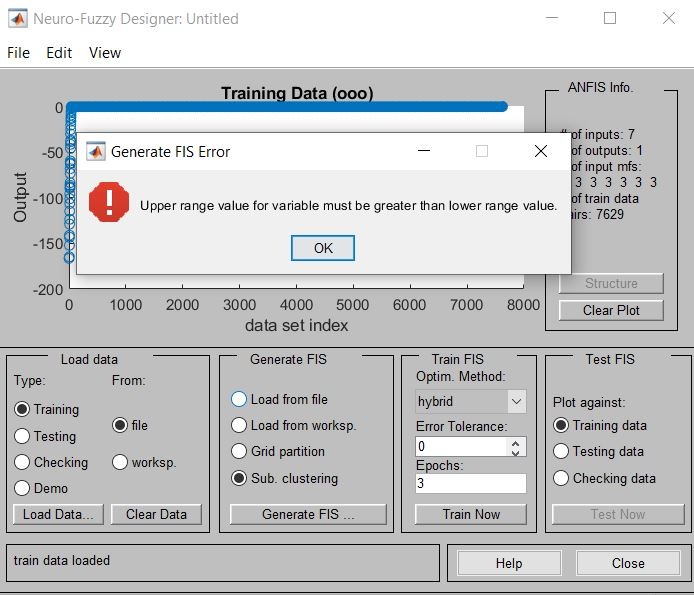Sale!

# Upper range value for the variable must be greater than the lower range

19

## Description

There is an error in ANFIS in MATLAB.

In this tutorial, you get familiar with solving this error in MATLAB.

“Error: Upper range value for the variable must be greater than the lower range”.

This error happens when you want to use genfis function in MATLAB.

```Error using fisvar/set.Range (line 218)
Upper range value for variable must be greater than lower range value.

Error in genfis2 (line 203)
var(i).Range = ranges(i,:);

Error in genfis (line 77)
fis = genfis2(Xin,Xout,args{:});```

This error can happen also in Neuro-Fuzzy Designer,In this tutorial, you will learn how to solve this error in MATLAB.